Article

# About Threads and Threadgroups

Learn how Metal organizes compute-processing workloads.

## Overview

Recall from Hello Compute that when you dispatch your compute pass, Metal executes your kernel function over a 1D, 2D, or 3D grid. Each point in the grid represents a single instance of your kernel function, referred to as a thread. For example, in image processing, the grid is typically a 2D matrix of threads—representing the entire image—with each thread corresponding to a single pixel of the image being processed.

Threads are organized into threadgroups that are executed together and can share a common block of memory. While sometimes kernel functions are designed so that threads run independently of each other, it's also common for threads in a threadgroup to collaborate on their working set.

### Identification of Threads by Position in Grid

Figure 1 shows how an image being processed by a compute kernel is divided into threadgroups and how each threadgroup is composed of individual threads. Each thread processes a single pixel.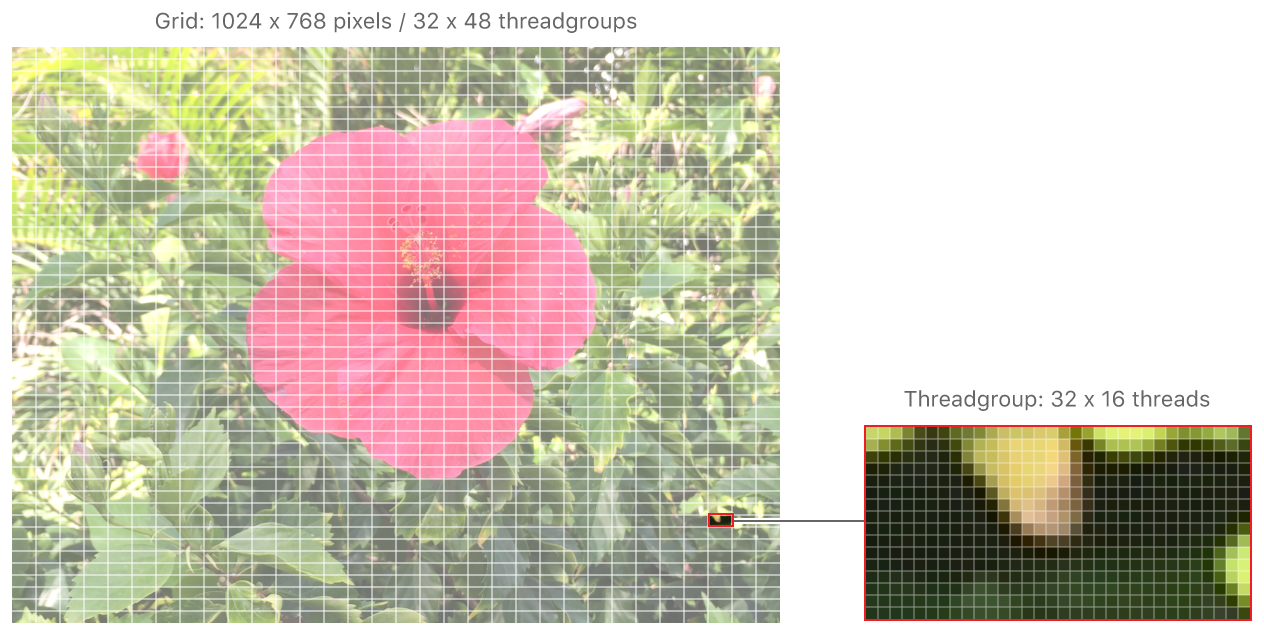Figure 1 A grid divided into threadgroups that are composed of individual threads.

A thread can be identified by its position in the grid; it's this unique position that allows your kernel function to do something different for each thread. The sample kernel function in Hello Compute, below, shows how a thread's position in the grid is passed into the function as a parameter. In this case, the parameter, `gid`, is a vector representing 2D coordinates and is used to both read from and write to a particular location in a texture.

`[[thread_position_in_grid]]` is an attribute qualifier. Attribute qualifiers, identifiable by their double square-bracket syntax, allow kernel parameters to be bound to resources and built-in variables, in this case the thread's position in the grid to the kernel function.

For example, given a grid of 16 x 16 threads partitioned into 2 x 4 threadgroups of 8 x 4 threads, a single thread (shown in Figure 2 in red) has a position in the grid of (9,10):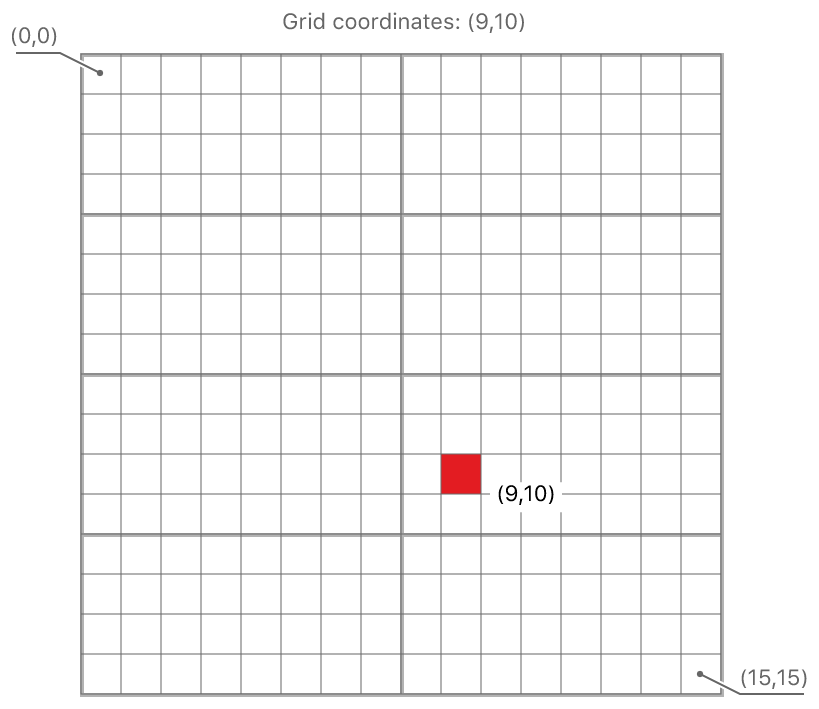Figure 2 The position of a single thread in a 16 x 16 grid.

### Identification of Threads by Position in Threadgroup

A thread's position in its threadgroup is also available as the attribute qualifier `[[thread_position_in_threadgroup]]`, and a threadgroup's position in the grid is available as `[[threadgroup_position_in_grid]]`.

Depending on the shape of the grid, these position attributes are either a scalar value, or a two- or three-element vector. In the case of a 2D grid, position attributes are two-element vectors, with the origin at the top left.

The thread identified in Figure 2 is in the threadgroup with a position in the grid of (1,2), and its position in that threadgroup is (1,2), as shown in Figure 3: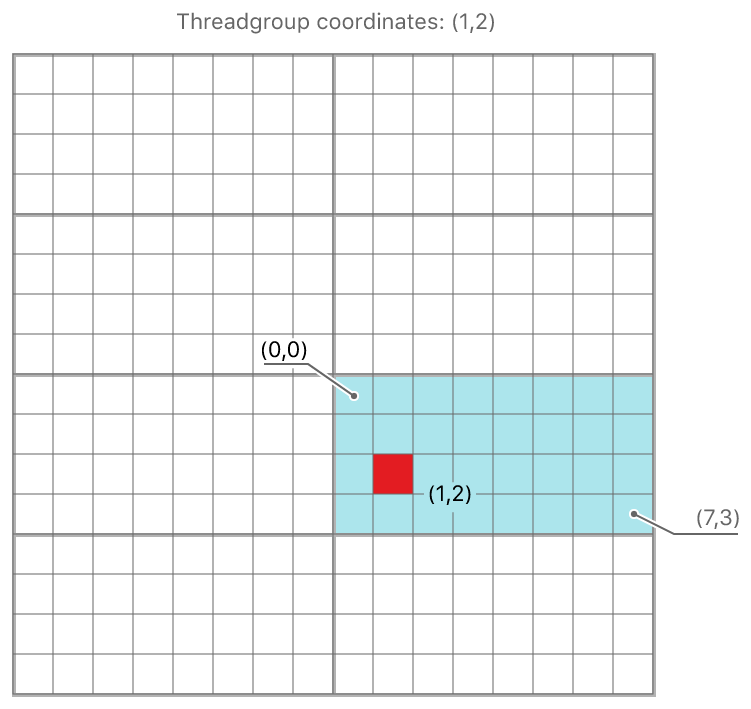Figure 3 The position of a single thread in a threadgroup.

Using the following code, you can also calculate a thread's position in the grid based on its position in its threadgroup and that threadgroup's size and position in the grid:

### SIMD Groups

The threads in a threadgroup are further organized into single-instruction, multiple-data (SIMD) groups, also known as warps or wavefronts, that execute concurrently. The threads in a SIMD group execute the same code. Avoid writing code that could cause your kernel function to diverge; that is, to follow different code paths. A typical example of divergence is caused by using an if statement. Even if a single thread in a SIMD group takes a different path from the others, all threads in that group execute both branches, and the execution time for the group is the sum of the execution time of both branches.

The division of threadgroups into SIMD groups is defined by Metal. It remains constant for the duration of a kernel's execution, across dispatches of a given kernel with the same launch parameters, and from one threadgroup to another within the dispatch.

The number of threads in a SIMD group is returned by the `threadExecutionWidth` of your compute pipeline state object. Attribute qualifiers allow you to access a SIMD group's scalar index within a threadgroup, and a thread's scalar index within a SIMD group:

`[[simdgroup_index_in_threadgroup]]`

The unique scalar index of a SIMD group in its threadgroup, also known as the lane ID.

`[[thread_index_in_simdgroup]]`

The unique scalar index of a thread in its SIMD group.

Although threadgroups can be multidimensional, SIMD groups are 1D. Therefore, a thread's position within a SIMD group is a scalar value for all threadgroup shapes. The SIMD group size remains constant and is unaffected by the threadgroup size.

For example, using the same 16 x 16 grid shown in Figure 2, with a thread execution width of 16, a single 8 x 4 threadgroup consists of 2 SIMD groups. Because a SIMD group contains 16 threads, each SIMD group constitutes 2 rows in the threadgroup: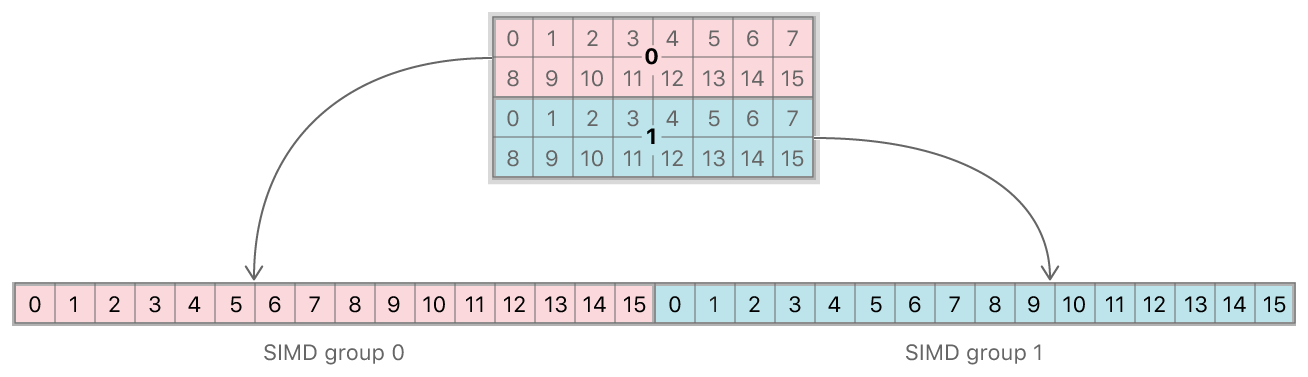Figure 4 A threadgroup composed of 2 SIMD groups.

The thread shown in red in Figure 5 has a `[[simdgroup_index_in_threadgroup]]` value of 1 and a `[[thread_index_in_simdgroup]]` value of 1: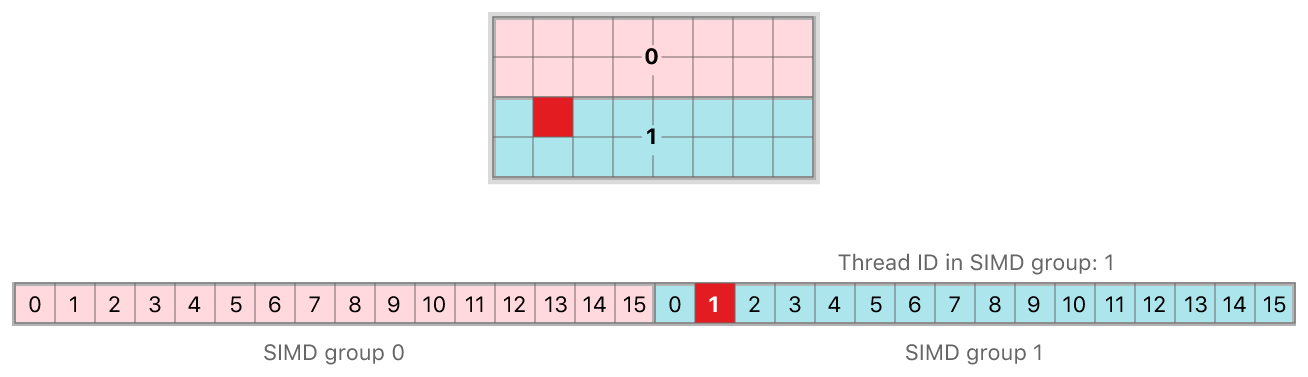Figure 5 The position of a single thread in a SIMD group.

## See Also

### Parallel Computation

Hello Compute

Demonstrates how to perform data-parallel computations using the GPU.

Calculating Threadgroup and Grid Sizes

Calculate the optimum sizes for threadgroups and grids when dispatching compute-processing workloads.

`MTLComputePipelineDescriptor`

An object used to customize how a new compute pipeline state object is compiled.

`MTLComputePipelineState`

An object that contains a compiled compute pipeline.

`MTLComputeCommandEncoder`

An object used to encode commands in a compute pass.